# Compare Dates In ExcelArticle byExcelMojo Team## What Is Comparing Two Dates In Excel?

The option to compare dates in Excel enables one to assess if two date values are the same or if one is greater or smaller than the other in a worksheet.

Users can compare two dates in Excel while reviewing the sales data from different periods and project progress in various phases.

For example, the table below contains a child’s date of birth, the current date, and a list of logical operators in Excel.

And the requirement is to compare the two date values using the specified logical operators and display the output in cells F4:F8.

Then, we can create a formula to compare dates in Excel cell F4 using the ‘=’ sign. And likewise, we can insert the compare dates formulas using the corresponding logical operators in the remaining target cells F5:F8.

The formula to compare dates in Excel cell F4 checks if the child’s birth date equals the current date.

So, Excel compares the sequential serial number equivalents of the date values, 44967 and 45083. And as the two numbers are not the same, the outcome is FALSE.

Next, the cell F5 formula verifies if the given child’s birth date is more recent than the current date. And as the first date’s equivalent serial number is smaller than the second date’s equivalent serial number, the formula output is FALSE.

Likewise, cells F6:F8 use the corresponding logical operators in cells E6:E8 to compare the two dates. And as the first date is older than the second date, Excel considers it smaller than the second date. So, the formula outputs in cells F6 and F8 are TRUE. And in cell F7, it is FALSE.

###### Key Takeaways
• The compare dates in Excel formulas enable one to check if the two specified dates are equal or which of the two is larger or smaller.
• Users can compare dates in a worksheet, even when the supplied dates are in different date formats. And the date comparison formulas are helpful while performing dates-based trend and pattern analysis in Excel.
• We can use logical operators, ‘=’, ‘>’, ‘<’, ‘>=’, ‘<=’, and ‘<>’, to compare date values in a worksheet.
• Using the logical operators in IF() and conditional formatting rules to compare dates yields practical results.

### How To Compare Two Dates In Excel?

We can compare two dates in Excel using the following two methods:

1. Compare If Two Dates are Equal or Not
2. Compare If Date is Greater or Smaller

#### #1 – Compare If Two Dates Are Equal Or Not

The steps to compare if the two specified dates are equal or not are as follows:

1. Enter the two dates in the required cells correctly.
2. Select a target cell to view the output.
3. Enter the ‘=‘ sign, the first date or cell reference to the first date, the ‘=‘ sign, and the second date or cell reference to the second date value.
4. Press Enter to view the two dates comparison.
##### Example #1

The table below lists items in column E and their order and delivery date data in columns F and G.

And the requirement is to determine if the items got delivered on the same day as ordered. Assume the target cells are H4:H8.

Then, we can compare dates in Excel columns F and G to check if the date values in each row in the two columns are equal and display the outcome in the corresponding target cells.

• Step 1: Choose cell H4, enter the following formula, and press Enter.

=F4=G4

• Step 2: Using the Excel fill handle, update the formula in the remaining target cells.

In the above compare dates in Excel columns example, we use the ‘=’ symbol to check the dates in each row of the two columns, F and G, are equal. And if the order and delivery dates are the same in a row, the formula output in the corresponding target cell is TRUE. Otherwise, the output is FALSE.

Please note Excel considers the sequential serial number equivalent of each date value, supplied in a formula, for calculations.

For example, the formula in cell H8 accepts the date values specified in cells F8 and G8. It then compares their serial number equivalents, 45052. And as the serial number equivalents of the two dates are the same, the output in cell H8 is TRUE.

Furthermore, it is best practice to supply a date value using a Excel DATE function. It will ensure the input dates are correct and valid.

For example, we can select cell H4 and enter the required formula using the DATE(), as shown below.

Likewise, we can select each target cell and enter the date comparison formula using the DATE() to obtain the required output.

The DATE() accepts three arguments, year, month, and day as the input to return the date value. And then, the formula checks if the two resulting dates are the same to return the output TRUE or FALSE accordingly.

##### Example #2

We shall see how to use the compare dates in Excel IF statement to check whether two given dates in different formats are equal.

The table below contains two date value sets in columns C and E. And columns B and D show the number formats of the dates in the corresponding rows in columns C and E, respectively.

The requirement is to check if the dates in each row of columns C and E are equal and display the outcome in the corresponding column F cells.

Then, we can use the compare dates in Excel IF statement in each target cell to obtain the required output.

=IF(C4=E4,”Dates Are Equal.”,”Dates Are Not Equal.”)

• Step 2: Implement the formula in cells F5:F8 using the fill handle.

The cells in rows 4 to 7 of columns C and E contain the same date value, 6/7/2023, but each in different Excel date format.

So, when the IF() in each target cell in the range F4:F7 checks whether the dates in the corresponding row of columns C and E are equal, the condition in the IF() holds. And thus, the function output in cells F4:F7 is the TRUE value, “Dates Are Equal.”.

However, in row 8, cell E8 contains a date’s sequential serial number. But the date is not 6/7/2023, and it is not equal to the cell C8 date value. And hence, the IF() condition does not hold in the target cell F8, and the function returns the FALSE value, “Dates Are Not Equal.”.

#### #2 – Compare If Date Is Greater Or Smaller

The steps to compare if a date is greater or smaller than the other are as follows:

1. Enter the two dates in the required cells correctly.
2. Select a target cell to view the output.
3. Enter the ‘=‘ sign, enter the first date value or cell reference to the first date value, the ‘>’ sign, and the second date value or cell reference to the second date value.

The above step will check if the first date is greater than the second. And if we have to check whether the first date is smaller than the second, use the ‘<’ sign instead of ‘>’ in the date comparison formula.

• Press Enter to view the two dates comparison output.
##### Example #1

The table below lists library books and their borrowed, returned and actual returned dates.

And the requirement is to check which books are overdue for return and those returned on time in columns F and G, respectively.

Then, we can apply the ‘>’ and ‘<‘ operators-based date comparison formulas in the target cells to achieve the required outcome.

• Step 1: Choose cell F3, enter the following formula, and press Enter.

=E3>D3

• Step 2: Enter the formula in the target cells F4:F8 using the fill handle.

The formulas in column F check if the actual return date is greater than the stipulated return date.

And if the actual return date, given in column E, falls after the return date or is more recent than the return date specified in column D.

Then, Excel considers it a greater date than the return date since the actual return date’s equivalent serial number will be greater than the return date’s serial number equivalent. And hence, the formula output is TRUE.

Otherwise, the output will be FALSE.

• Step 3: Choose cell G3, enter the following formula, and press Enter.

=E3<D3

• Step 4: Enter the formula in the target cells G4:G8 using the fill handle.

The formula in each target cell in column G checks if the actual return date is smaller than the stipulated return date.

And if the actual return date, given in column E, falls before or is older than the return date specified in column D.

Then, Excel considers it a smaller date than the return date since the actual return date’s serial number equivalent will be smaller than the return date’s serial number equivalent. And hence, the formula output is TRUE.

Otherwise, the output will be FALSE.

##### Example #2

We shall see an example of compare dates in Excel conditional formatting.

The table below contains a set of reports, their deadline, and actual submission dates.

The requirement is to highlight the submission dates of those reports in column F that have breached the submission deadline.

Then, we can select cells F2:F7 and apply compare dates in Excel conditional formatting to highlight the required cells.

And then, select New Rule from the drop-down list to access the New Formatting Rule window.

Otherwise, we can press Alt + H + L + N to open the New Formatting Rule window.

Alternatively, we can use the shortcut keys, Alt + O + D, to open the Conditional Formatting Rules Manager window. And then, click the New Rule button to open the New Formatting Rule window.

• Step 2: Select the last rule type to enter the formula for deciding the cells to format.
• Step 3: Enter the required formula in the field inside the Edit the Rule Description section as the conditional formatting rule.

=F2>\$E\$2

And then click Format.

The Excel Format Cells window will open, where we can set the format we wish to apply to the required cells.

So, click the Font tab to open it and select the Font Style as Bold.

And then, click the Fill tab to open it and choose the required color from the given Background Color options.

Click OK in the Format Cells window to close it.

Click OK in the New Formatting Rule window to close it and achieve the following output.

The conditional formatting rule will compare each cell date value in the range F2:F7 with the date value in cell E2 to check whether it is greater than the date value in cell E2.

And if the column F cell date value’s serial number equivalent is greater than the cell E2 date value’s serial number equivalent, the condition holds. And the corresponding cell gets highlighted with the chosen format.

### Important Things To Note

• When we enter formulas to compare dates in Excel, the program considers the sequential serial number equivalent of each date value for calculations.
• Use the DATE excel function to enter date values in the source dataset or the formulas. It will help avoid supplying incorrect and invalid date values for date comparisons.
• Sometimes a text may appear like a date value. So, before comparing dates, confirm the supplied values are in the required date format to avoid the formula returning errors or incorrect values.

1. How do you compare dates in Excel and highlight differences?

You can compare dates in Excel and highlight differences using the logical operators and the DATEDIF() in the IF().

For example, the table below lists tasks and their start and end dates.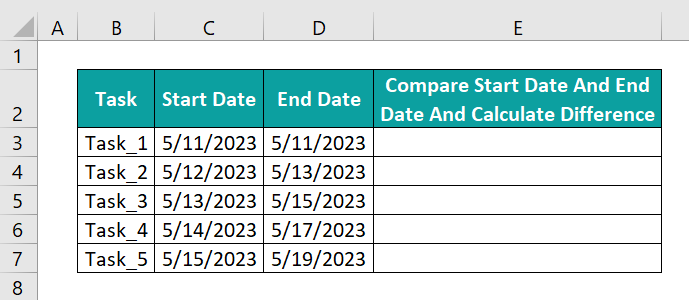And the requirement is to compare the start and end dates and highlight the difference between the two date values for each task in column E.

Then, the steps are as follows:

• Step 1: Select cell E3, enter IF(), and press Enter.
=IF(C3=D3,DATEDIF(C3,D3,”d”),IF(C3<D3,DATEDIF(C3,D3,”d”),”Invalid Data – End Date is before Start Date”))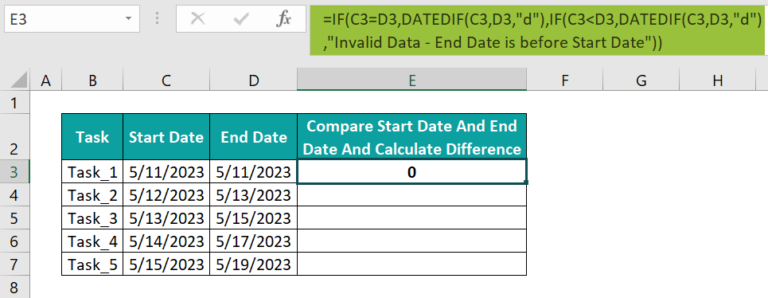• Step 2: With the help of the fill handle, update the formula in the remaining target cells.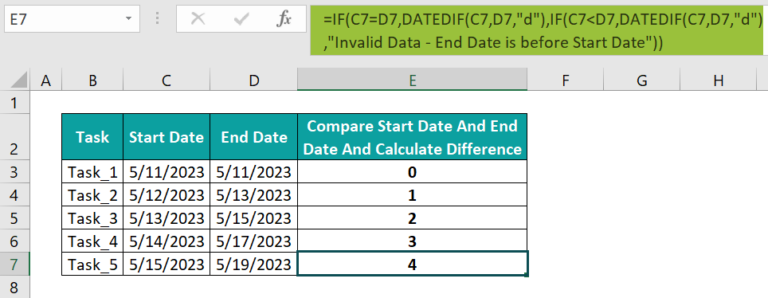We shall see the cell E7 formula to understand how it works.

First, the outer IF() condition checks if the dates in cells C7 and D7 are equal. And as they are not the same, the FALSE value gets considered.

So, the inner IF() executes. It checks if the cell C7 date value is smaller or older than the cell D7 date value. And as the condition holds, the TRUE value gets considered.

Thus, the inner DATEDIF() gets executed. And as the format specified in the DATEDIF() is “d”, it returns the number of full days between the quoted start and end dates, 4, as the IF() output.

2. How do I compare two dates in Excel without time?

You can compare two dates in Excel without time using the logical operators and the INT().
For example, the table below shows the admission date and time entries of a list of applicants and the admission cut-off date at a college.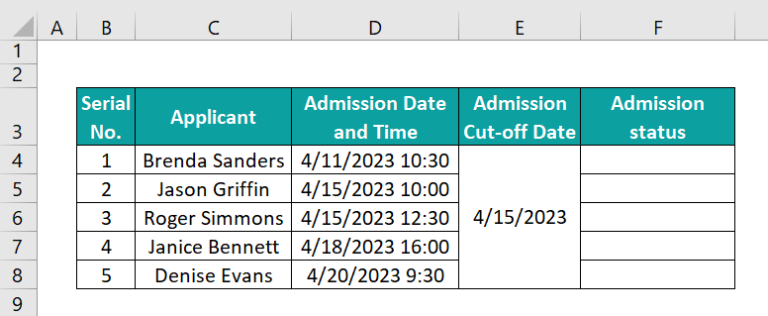And the requirement is to determine the admission status of each applicant in column F.

Then, the steps are as follows:

• Step 1: Select cell F4, enter the following formula, and press Enter.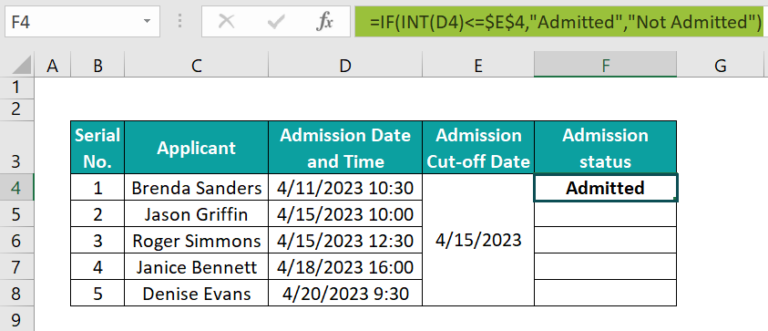• Step 2: Enter the formula in the remaining target cells using the fill handle.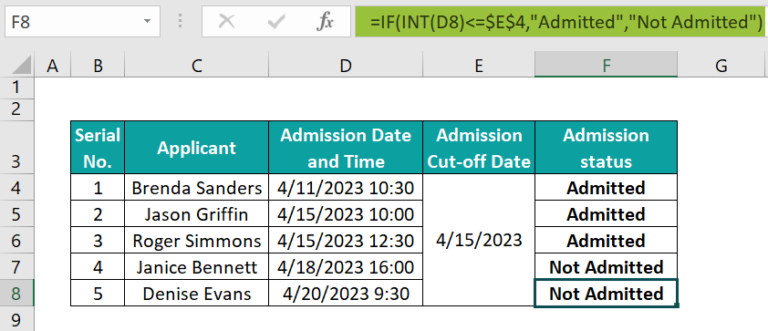Let us check the cell F8 formula to know how it works.

First, the INT() accepts the date and time value specified in cell D8. And it rounds down the sequential serial number equivalent of the given date and time value, 45036.3958333333, to the nearest integer, 45036.

Next, the IF() condition checks if the INT() output, 45036, is less than or equal to the sequential serial number equivalent of the date value in cell E4, 45031. And as the condition does not hold, the IF() output is the FALSE value, “ Not Admitted”.

3. Why can’t I compare dates in Excel?

You can’t compare dates in Excel, perhaps due to the following reasons:
You entered invalid date values.
The supplied dates have incorrect date formats.
The dates comparison formula is incorrect.
You entered the cell reference to one date and the second date directly in the formula.